# Symmetric group

The group of all permutations (self-bijections) of a setwith the operation of composition (see Permutation group). The symmetric group on a setis denoted by. For equipotentand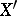the groupsand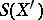are isomorphic. The symmetric group of a finite setis denoted by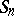. Every abstract group is isomorphic to a subgroup of the symmetric groupof some set(Cayley's theorem).

Letbe a finite set. Every permutationoncan be uniquely described as a product of disjoint cycles (the (disjoint) cycle decomposition of a permutation); the sequence of integers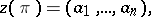where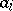is the number of cycles of lengthof, is called the cycle type (or cycle index) of. Two permutationsandare conjugate inif and only if they have the same cycle type. Permutations with cycle type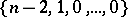are called transpositions; they form a system of generators for. The set of transpositions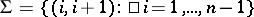is a minimal set of generators for. In general, a set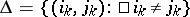generatesif the graph withas set of vertices and the pairs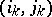as edges is a tree . The number of such generating sets is.

The alternating groupis a normal subgroup of. If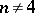,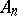is the unique non-trivial proper normal subgroup, and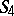contains only one other non-trivial normal subgroup — the Klein four-group: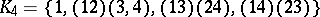. Forthe groupis solvable, but forit is not solvable andis a simple non-Abelian group. Hölder's theorem: For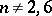, the groupis complete (see Complete group). The groupis commutative, and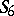has an outer automorphism of order 2.

All maximal intransitive and imprimitive subgroups inare known. For each partition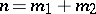,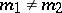, the only maximal intransitive subgroups inare the subgroups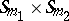. The only transitive imprimitive maximal subgroups inare the wreath products (cf. Wreath product) of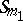with(for every decomposition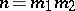). The primitive maximal subgroups inhave not yet been described (1992), but certain infinite series of them are known. For example,acts naturally on the set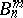of all subsets ofelements of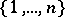; for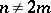this determines a primitive group of permutations of. It is maximal in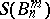if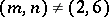,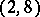,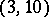,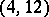,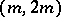,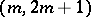(see ). Another series is obtained by considering the group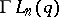of semi-linear transformations of the-dimensional vector space over the finite field ofelements. This group defines a primitive group of permutations of the Grassmann manifold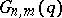,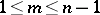, which is maximal infor.

Letbe an infinite set. The group of all permutations ofthat move only a finite number of elements ofis called the finitary, or restricted, symmetric group on, and is denoted by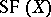; its subgroup of even permutations is called the finite, or restricted, alternating group on, and is denoted by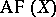. The subgroupsandare normal in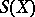. More generally, letbe the cardinality ofand let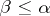be an infinite cardinal; the set of permutations ofwhich move at mostelements ofis a subgroup of, denoted by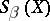. Along withand, the groupsare normal subgroups infor all, and in fact there are no other non-trivial normal subgroups in(the Schreier–Ulam–Baer theorem, see ).

See also the references to Permutation of a set.